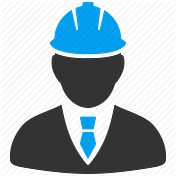# Model That Will Recognize Characters on Number Plate

By- Sandeep Kumar Patel

# Steps involved in License Plate Recognition

1. License Plate Detection: The first step is to detect the License plate from the car. We will use the contour option in OpenCV to detect for rectangular objects to find the number plate. The accuracy can be improved if we know the exact size, color and approximate location of the number plate. Normally the detection algorithm is trained based on the position of camera and type of number plate used in that particular country. This gets trickier if the image does not even have a car, in this case we will an additional step to detect the car and then the license plate.

2. Character Segmentation: Once we have detected the License Plate we have to crop it out and save it as a new image. Again this can be done easily using OpenCV.

3. Character Recognition: Now, the new image that we obtained in the previous step is sure to have some characters (Numbers/Alphabets) written on it. So, we can perform OCR (Optical Character Recognition) on it to detect the number

# 1. License Plate Detection

Let’s take a sample image of a car and start with detecting the License Plate on that car. We will then use the same image for Character Segmentation and Character Recognition as well. If you want to jump straight into the code without explanation then you can scroll down to the bottom of this page

# OpenCV: Automatic License/Number Plate Recognition (ANPR) with Python

My first run-in with ANPR was about six years ago.

After a grueling three-day marathon consulting project in Maryland, where it did nothing but rain the entire time, I hopped on I-95 to drive back to Connecticut to visit friends for the weekend.

# Python Code Sandeep.py-

print(“Number input image…”,)

cv2.imshow(“input”,img)

if cv2.waitKey(0) & 0xff == ord(‘q’):

pass

img2 = cv2.GaussianBlur(img, (3,3), 0)

img2 = cv2.cvtColor(img2, cv2.COLOR_BGR2GRAY)

img2 = cv2.Sobel(img2,cv2.CV_8U,1,0,ksize=3)

_,img2 = cv2.threshold(img2,0,255,cv2.THRESH_BINARY+cv2.THRESH_OTSU)

element = cv2.getStructuringElement(shape=cv2.MORPH_RECT, ksize=(17, 3))

morph_img_threshold = img2.copy()

cv2.morphologyEx(src=img2, op=cv2.MORPH_CLOSE, kernel=element, dst=morph_img_threshold)

num_contours, hierarchy= cv2.findContours(morph_img_threshold,mode=cv2.RETR_EXTERNAL,method=cv2.CHAIN_APPROX_NONE)

cv2.drawContours(img2, num_contours, -1, (0,255,0), 1)

for i,cnt in enumerate(num_contours):

min_rect = cv2.minAreaRect(cnt)

if ratio_and_rotation(min_rect):

x,y,w,h = cv2.boundingRect(cnt)

plate_img = img[y:y+h,x:x+w]

print(“Number identified number plate…”)

cv2.imshow(“num plate image”,plate_img)

if cv2.waitKey(0) & 0xff == ord(‘q’):

pass

if(isMaxWhite(plate_img)):

clean_plate, rect = clean2_plate(plate_img)

if rect:

fg=0

x1,y1,w1,h1 = rect

x,y,w,h = x+x1,y+y1,w1,h1

# cv2.imwrite(“clena.png”,clean_plate)

plate_im = Image.fromarray(clean_plate)

text = tess.image_to_string(plate_im, lang=’eng’)

print(“Number Detected Plate Text : “,text)

# import tkinter as tk

from tkinter import filedialog

from tkinter import *

from PIL import ImageTk, Image

from tkinter import PhotoImage

import numpy as np

import cv2

import pytesseract as tess

def clean2_plate(plate):

gray_img = cv2.cvtColor(plate, cv2.COLOR_BGR2GRAY)

_, thresh = cv2.threshold(gray_img, 110, 255, cv2.THRESH_BINARY)

num_contours,hierarchy = cv2.findContours(thresh.copy(),cv2.RETR_EXTERNAL, cv2.CHAIN_APPROX_NONE)

if num_contours:

contour_area = [cv2.contourArea(c) for c in num_contours]

max_cntr_index = np.argmax(contour_area)

max_cnt = num_contours[max_cntr_index]

max_cntArea = contour_area[max_cntr_index]

x,y,w,h = cv2.boundingRect(max_cnt)

if not ratioCheck(max_cntArea,w,h):

return plate,None

final_img = thresh[y:y+h, x:x+w]

return final_img,[x,y,w,h]

else:

return plate,None

def ratioCheck(area, width, height):

ratio = float(width) / float(height)

if ratio < 1:

ratio = 1 / ratio

if (area < 1063.62 or area > 73862.5) or (ratio < 3 or ratio > 6):

return False

return True

def isMaxWhite(plate):

avg = np.mean(plate)

if(avg>=115):

return True

else:

return False

def ratio_and_rotation(rect):

(x, y), (width, height), rect_angle = rect

if(width>height):

angle = -rect_angle

else:

angle = 90 + rect_angle

if angle>15:

return False

if height == 0 or width == 0:

return False

area = height*width

if not ratioCheck(area,width,height):

return False

else:

return True

top=tk.Tk()

top.geometry(‘900x700’)

top.title(‘Number Plate Recognition’)

top.iconphoto(True, PhotoImage(file=”/home/shivam/Dataflair/Keras Projects_CIFAR/GUI/logo.png”))

img = ImageTk.PhotoImage(Image.open(“logo.png”))

top.configure(background=’#CDCDCD’)

label=Label(top,background=’#CDCDCD’, font=(‘arial’,35,’bold’))

# label.grid(row=0,column=1)

sign_image = Label(top,bd=10)

plate_image=Label(top,bd=10)

def classify(file_path):

res_text=

res_img=

img2 = cv2.GaussianBlur(img, (3,3), 0)

img2 = cv2.cvtColor(img2, cv2.COLOR_BGR2GRAY)

img2 = cv2.Sobel(img2,cv2.CV_8U,1,0,ksize=3)

_,img2 = cv2.threshold(img2,0,255,cv2.THRESH_BINARY+cv2.THRESH_OTSU)

element = cv2.getStructuringElement(shape=cv2.MORPH_RECT, ksize=(17, 3))

morph_img_threshold = img2.copy()

cv2.morphologyEx(src=img2, op=cv2.MORPH_CLOSE, kernel=element, dst=morph_img_threshold)

num_contours, hierarchy= cv2.findContours(morph_img_threshold,mode=cv2.RETR_EXTERNAL,method=cv2.CHAIN_APPROX_NONE)

cv2.drawContours(img2, num_contours, -1, (0,255,0), 1)

for i,cnt in enumerate(num_contours):

min_rect = cv2.minAreaRect(cnt)

if ratio_and_rotation(min_rect):

x,y,w,h = cv2.boundingRect(cnt)

plate_img = img[y:y+h,x:x+w]

print(“Number identified number plate…”)

res_img=plate_img

cv2.imwrite(“result.png”,plate_img)

if(isMaxWhite(plate_img)):

clean_plate, rect = clean2_plate(plate_img)

if rect:

fg=0

x1,y1,w1,h1 = rect

x,y,w,h = x+x1,y+y1,w1,h1

plate_im = Image.fromarray(clean_plate)

text = tess.image_to_string(plate_im, lang=’eng’)

res_text=text

if text:

break

label.configure(foreground=’#011638', text=res_text)

plate_image.configure(image=im)

plate_image.image=im

plate_image.pack()

plate_image.place(x=560,y=320)

def show_classify_button(file_path):

classify_b.configure(background=’#364156', foreground=’white’,font=(‘arial’,15,’bold’))

classify_b.place(x=490,y=550)

try:

sign_image.configure(image=im)

sign_image.image=im

label.configure(text=’’)

show_classify_button(file_path)

except:

pass

sign_image.pack()

sign_image.place(x=70,y=200)

label.pack()

label.place(x=500,y=220)

top.mainloop()

Thank For Your Time….!!!!

--

--## Sandeep Kumar Patel

Enthusiastic, aspirant in artificial intelligence, machine learning Research is formalized curiosity. It is poking and prying with a purpose.